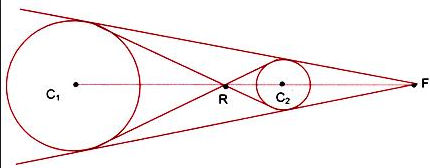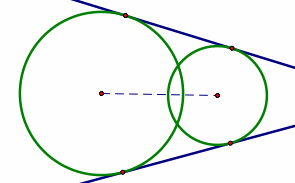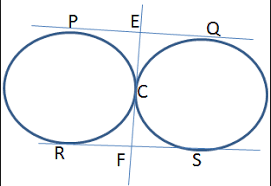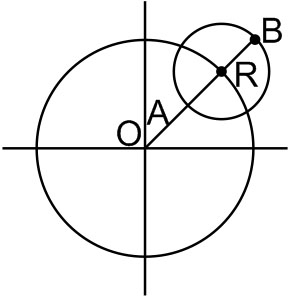# The centres of a set of circles, each of radius 3, lie on the circle The locus of any point in the set is      Option 1) Option 2) Option 3) Option 4)

As we learnt in

Common tangents of two circle -

Where two circle neither intersect nor touch each other, there are 4 common tangents.Two are transverse and two are direct common tangents.

- whereinand

Common tangents of two circle -

When they intersect, there are two common tangents, both of them being direct.

- whereinand

Common tangents of two circles -

When two circles touch  each other externally, there are three common tangents, two of them are direct.

- whereinWe should haveSo,

Option 1)

This option is correct

Option 2)

This option is incorrect

Option 3)

This option is incorrect

Option 4)

This option is incorrect

Exams
Articles
Questions